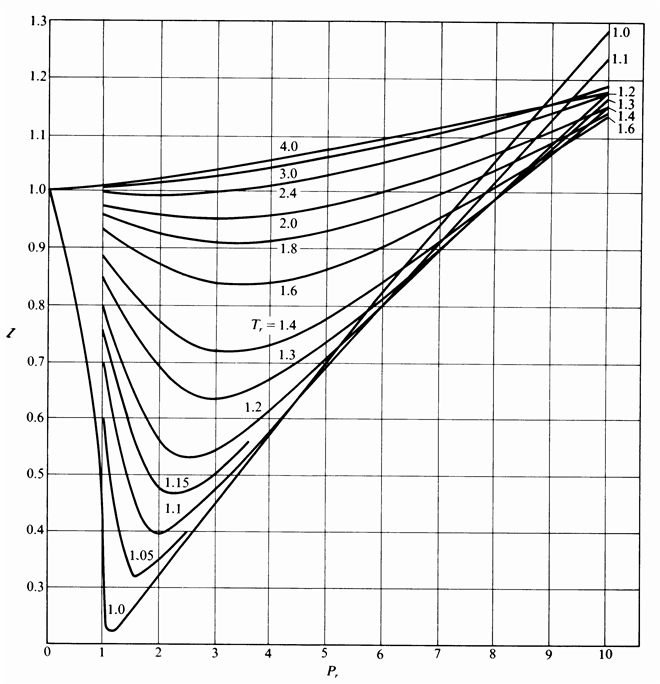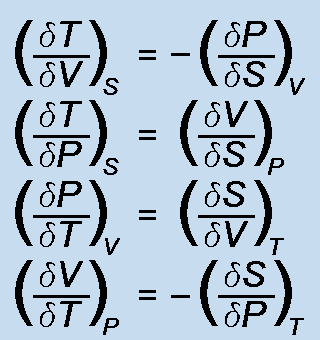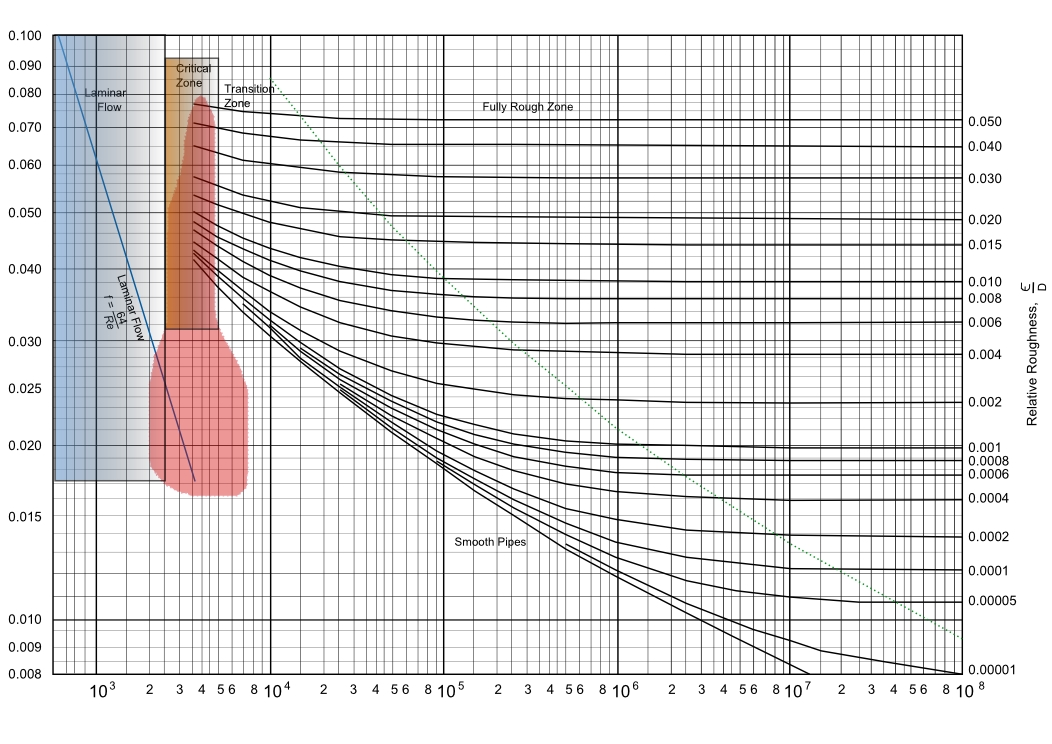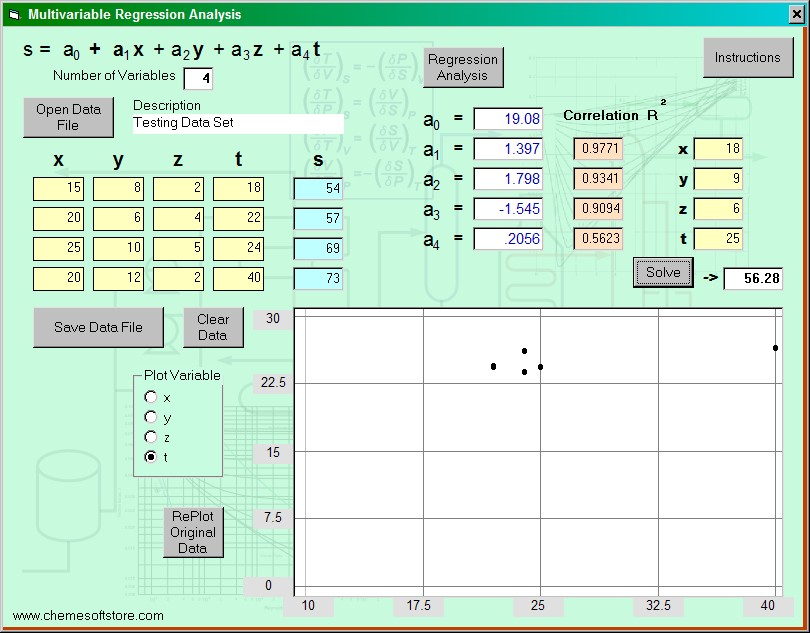### ChemE Soft StoreCaCO3 -> CaO + CO2
NaCl + NH3 + CO2 + H2O -> NaHCO3 + NH4Cl
2 NaHCO3 -> Na2 CO3 + H2O + CO2
CaO + H2 O -> Ca(OH)2
Ca(OH)2 + 2 NH4 Cl -> CaCl2 + 2 NH3 + 2 H2O

## Multivariable Regression

Multivariable regression analysis of up to four independent variables.  Each independent variable is solved, plotted, and a correlation coefficient calculated. Plots are normalized for each variable to show linearity, or goodness of fit.#### Features:

• Up to four independent variables can be entered and analyzed
• Up to 200 data lines can be input for regression analysis
• Correlation coefficients for each independent variable are calculated
• Plots of each independent variable are presented for linearity
• New values of S can be calculated from input variable data
• Instructions for the software application is presented